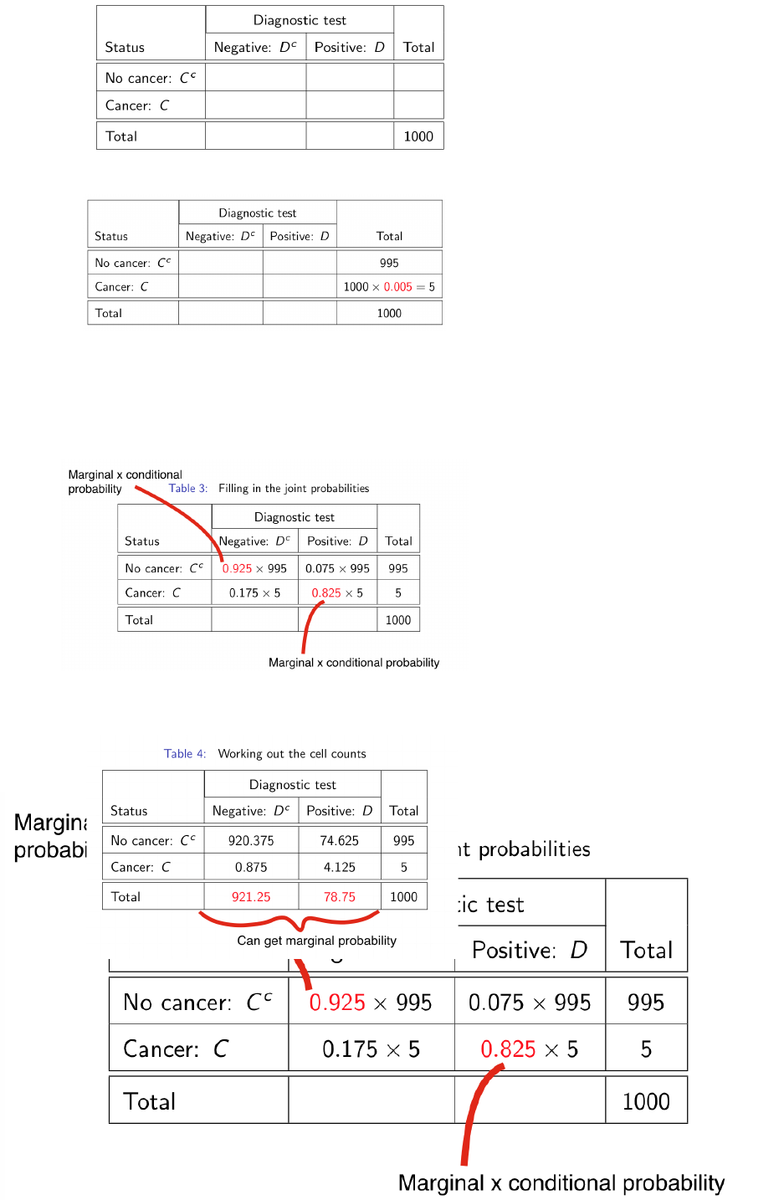Class Notes (1,100,000)
US (480,000)
UPenn (2,000)
STAT (70)
Lecture 9

# STAT 101 Lecture Notes - Lecture 9: Contingency TablePremium

Department
Statistics
Course Code
STAT 101
Professor
Richard Waterman
Lecture
9

This preview shows page 1. to view the full 5 pages of the document.Stat 101 - Introduction to Business Statistics - Lecture 9: Bayes Theorem
Cancer Example
You have a cancer screening test. It comes back positive. How much should you worry?
You want to find the probability of cancer given a positive screening test
**** Ultimately we wish to find P(C|D).
Required facts:
The probability a test comes back negative given no cancer (specificity).
1 - false positive rate
The probability a test comes back positive given cancer (sensitivity).
1 - false negative rate
The probability of cancer (with no conditioning). (marginal probability)
Define variables
Call C the event that cancer is present.
Call Cc the event cancer is not present.
Call D the event the diagnostic test comes back positive.
call Dc the event the diagnostic test comes back negative.
Available information:
Specificity = P(Dc |Cc )=0.925.
Sensitivity = P(D|C)=0.825.
Population prevalence = P(C)=0.005.
**Note: The key insight is that these conditional probabilities will allow us to
propagate the marginal probabilities back into the cells of the contingency table.
Now, construct a contingency table
Step 1: Work out cell counts (assume here that 1000 people have test done)

Unlock to view full version

Subscribers Only

Only page 1 are available for preview. Some parts have been intentionally blurred.

Subscribers OnlyStep 2: fill in the margin based on the prevalence of cancer
Step 3: Use the conditional probabilities to propagate the marginal probabilities to
the cells. Remember “joint equals marginal times conditional”
Step 4: Work out the numbers, including second margin